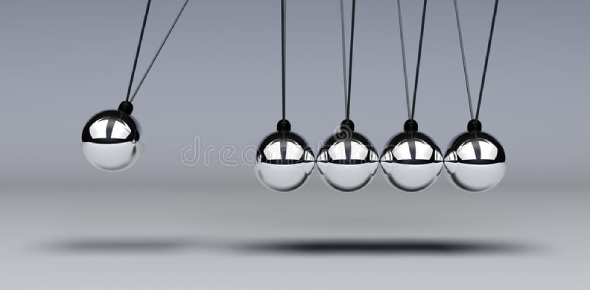# Newton's Laws Of Motion Test Quiz! Trivia

10 Questions | Total Attempts: 1489Settings.

• 1.
Which of the following is Newton's First Law of Motion?
• A.

For every action there is an equal and opposite reaction force.

• B.

The same force on a large mass and a small mass will accelerate the smaller mass more.

• C.

An object moving will keep moving and an object at rest will stay at rest unless a force is applied.

• 2.
Which of the following is Newton's Second Law of Motion?
• A.

For every action there is an equal and opposite reaction force.

• B.

The same force on a large mass and a small mass will accelerate the smaller mass more.

• C.

An object moving will keep moving and an object at rest will stay at rest unless a force is applied.

• 3.
Which of the following is Newton's Third Law of Motion?
• A.

For every action there is an equal and opposite reaction force.

• B.

The same force on a large mass and a small mass will accelerate the smaller mass more.

• C.

An object moving will keep moving and an object at rest will stay at rest unless a force is applied.

• 4.
A magician pulls a table clothe out from under the plates and silverware without pulling everything onto the floor. This is an example of which Law of Motion?
• A.

First Law of Motion

• B.

Second Law of Motion

• C.

Third Law of Motion

• 5.
A magician pulls a table clothe out from under the plates and silverware without pulling everything onto the floor. This is because:
• A.

Objects in motion remain in motion unless a force is applied.

• B.

Objects at rest remain at rest unless a force is applied.

• C.

The same force accelerates objects with less mass more than objects with more mass.

• D.

For every action there is an equal and opposite reaction.

• 6.
You are in a car that skids to a sudden stop. You body is flung forward into the back of the seat in front of you. This happened because
• A.

Objects in motion remain in motion unless a force is applied.

• B.

Objects at rest remain at rest unless a force is applied.

• C.

The same force accelerates objects with less mass more than objects with more mass.

• D.

For every action there is an equal and opposite reaction.

• 7.
While playing a kick ball game, you can kick long home runs with the rubber ball you are using. Unfortunately, the ball pops. Luckily, someone has a soccer ball. You notice that when you kick the heavier soccer ball, the ball does not travel as quickly and you can only make it to second base. This is because
• A.

Objects in motion remain in motion unless a force is applied.

• B.

Objects at rest remain at rest unless a force is applied.

• C.

The same force accelerates objects with less mass more than objects with more mass.

• D.

For every action there is an equal and opposite reaction.

• 8.
Which of the following is NOT an example of acceleration?
• A.

Turning the corner of the street while riding your bike.

• B.

Jogging steadily along a hiking trail.

• C.

Slowing down to stop for a stop light.

• D.

Jumping out of bed.

• 9.
When you sit your books on the table, the book do not break the table because
• A.

The table destroys the gravity that is pulling on the books.

• B.

The table pushes harder than the gravity pulling down the books.

• C.

Friction stops the books from moving.

• D.

The table pushes up on the books as hard as gravity pulls down on the books.

• 10.
A sail boat is sailing along the lake. Which of the following describes the force pair that helps move the sail boat?            Action Force         :          Reaction Force
• A.

Air Resistance on wind : Wind on air resistance

• B.

Wind blowing on the water : Water blowing on wind

• C.

Wind pushing on sail : Sail pushing on wind

• D.

Sail pushing on wind : Wind pushing on sail

Related TopicsBack to top